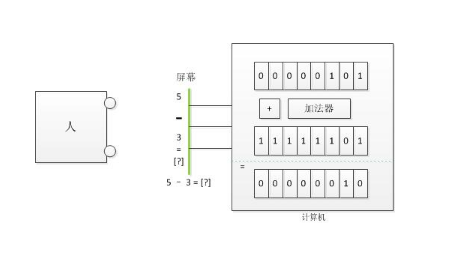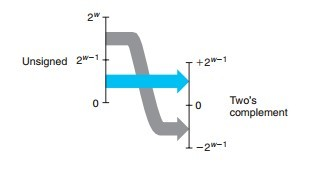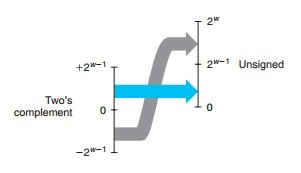# 补码

## 参考## 时钟

16 - 11 = 5，即补码就是 5。

### 模 mod

• 一个包含两个 bit 的存储单位，它可以表示`00`，加一变成`01`，加一变成`10`，加一变成`11`，再加一又变成了`00`。即该计数系统的`Mod``4`

• 一个时钟显示十二个小时，时针旋转一周后回到原来的状态，即该系统的`Mod``12`

### 变减为加

`Mod = 16`

`a=3，b=-4`

``````a + b = 3 - 4 = -1;

a + b = a + b + Mod;

a + b + Mod = a + ( Mod + b) = 3 + ( Mod - 4 ) = 15

-1 = 15; # 在计数系统中 -1 和 15 等价

Mod - 4 = 12;``````

`4``Mod - 4` 互为关于`Mod`的补数，`被减数 - 减数` 等价于`被减数 + Mod - 减数`，即 `被减数 + 减数的补数`

### 使用补码运算

16 - 13，模为 32，使用补码运算该算式？

``(16 + ( 32 - 13 ) ) % 32 = 35 % 32 = 3``

### 约定的正负号

``````1111 = 15    0000 = 0
1110 = 14    0001 = 1
1101 = 13    0010 = 2
1100 = 12    0011 = 3
1011 = 11    0100 = 4
1010 = 10    0101 = 5
1001 = 9     0110 = 6
1000 = 8     0111 = 7``````

``````1111 = -1    0000 = 0
1110 = -2    0001 = 1
1101 = -3    0010 = 2
1100 = -4    0011 = 3
1011 = -5    0100 = 4
1010 = -6    0101 = 5
1001 = -7    0110 = 6
1000 = -8    0111 = 7``````

Mod 为 16,即`10000`,对于`1 - 1 = 0`这个等式，等价于`1 + (-1) = 0`；即`0001（二进制） + （-1）= 0000（二进制）`，那么`（-1）= 0000 - 0001`，向高位借 1 位后，减得`（-1）= 1111（二进制）`，所以计算机中使用`1111`来表示以及存储`-1`

## 无符号数``````#include <stdio.h>
int main(){
char t = 0xFF;
unsigned char u = 0xFF;
printf("t=%d u=%u\n",t,u);
printf("t2u=%u u2t=%d\n",(unsigned char)t,(char)u);
}
// t=-1 u=255
// t2u=255 u2t=-1``````